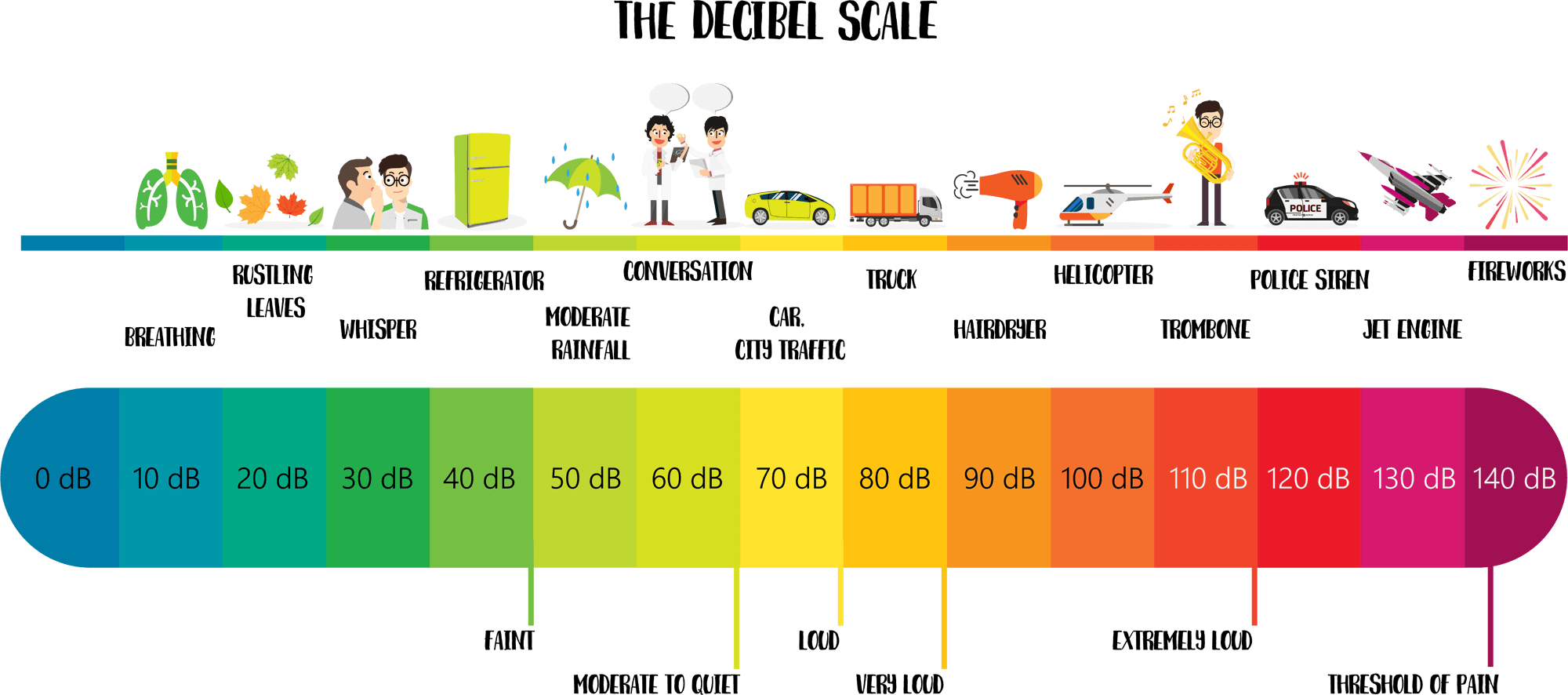BUY THE YEAR 9 PHYSICS WORKBOOK

## Sound Intensity and the Decibel Scale

• The decibel scale is a more objective way of measuring the loudness (intensity) of sound.
It uses the units decibels, which have the symbol dB.
• The scale begins at 0 dB. This doesn’t mean there is no sound, but instead represents the quietest sound that the human ear can detect, known as the threshold of hearing. The decibel scale uses this sound level as its zero reference point.
• The decibel scale is a logarithmic scale. This means that for every 10 dB increase, there is a 10-times increase in sound intensity.
Examples
• A 30 dB sound is 10 times more intense than a 20 dB sound.
• A 40 dB sound is 100 times more intense than a 20 dB sound.
• A 50 dB sound is 1000 times more intense than a 20 dB sound.
• Exposure to sound levels above 90 dB can cause permanent damage to hearing, with the consequences becoming more severe with longer exposure.
• The decibel level and intensity level of typical sounds are shown in the table below.The decibel (dB) scale is a way of measuring the loudness of sounds.